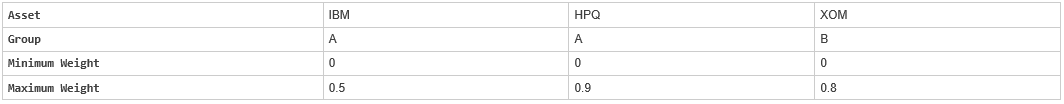# portcons

Portfolio constraints

## Syntax

``ConSet = portcons(ConstType,consttype_values)``

## Description

As an alternative to `portcons`, use the Portfolio object (`Portfolio`) for mean-variance portfolio optimization. This object supports gross or net portfolio returns as the return proxy, the variance of portfolio returns as the risk proxy, and a portfolio set that is any combination of the specified constraints to form a portfolio set. For information on the workflow when using Portfolio objects, see Portfolio Object Workflow.

example

````ConSet = portcons(ConstType,consttype_values)` generates a matrix of constraints, using linear inequalities, for a portfolio of asset investments. The inequalities are of the type ```A*Wts' <= b```, where `Wts` is the matrix of weights. The matrix `ConSet` is defined as ```ConSet = [A b]```.```

## Examples

collapse all

Constrain a portfolio of three assets:```NumAssets = 3; PVal = 1; % Scale portfolio value to 1. AssetMin = 0; AssetMax = [0.5 0.9 0.8]; GroupA = [1 1 0]; GroupB = [0 0 1]; AtoBmax = 1.5 % Value of assets in Group A at most 1.5 times value ```
```AtoBmax = 1.5000 ```
``` % in group B. ConSet = portcons('PortValue', PVal, NumAssets,'AssetLims',... AssetMin, AssetMax, NumAssets, 'GroupComparison',GroupA, NaN,... AtoBmax, GroupB)```
```ConSet = 9×4 1.0000 1.0000 1.0000 1.0000 -1.0000 -1.0000 -1.0000 -1.0000 1.0000 0 0 0.5000 0 1.0000 0 0.9000 0 0 1.0000 0.8000 -1.0000 0 0 0 0 -1.0000 0 0 0 0 -1.0000 0 1.0000 1.0000 -1.5000 0 ```

For instance, one possible solution for portfolio weights that satisfy the constraints is 30% in IBM, 30% in HPQ, and 40% in XOM.

## Input Arguments

collapse all

Constraint type, specified as a character vector defined as follows:

Constraint Type

Description

Values

`'Default'`

All allocations are >= 0; no short selling allowed. Combined value of portfolio allocations normalized to 1.

`NumAssets` (required). Scalar representing number of assets in portfolio.

`'PortValue'`

Fix total value of portfolio to `PVal`.

`PVal` (required). Scalar representing total value of portfolio.

```NumAssets ```(required). Scalar representing number of assets in portfolio. See `pcpval`.

`'AssetLims'`

Minimum and maximum allocation per asset.

`AssetMin` (required). Scalar or vector of length `NASSETS`, specifying minimum allocation per asset.

`AssetMax` (required). Scalar or vector of length `NASSETS`, specifying maximum allocation per asset.

`NumAssets` (optional). See `pcalims`.

`'GroupLims'`

Minimum and maximum allocations to asset group.

`Groups` (required). `NGROUPS`-by-`NASSETS` matrix specifying which assets belong to each group.

`GroupMin` (required). Scalar or a vector of length `NGROUPS`, specifying minimum combined allocations in each group.

`GroupMax` (required). Scalar or a vector of length `NGROUPS`, specifying maximum combined allocations in each group.

`'GroupComparison'`

Group-to-group comparison constraints.

`GroupA` (required). `NGROUPS`-by-`NASSETS` matrix specifying first group in the comparison.

`AtoBmin` (required). Scalar or vector of length `NGROUPS` specifying minimum ratios of allocations in `GroupA` to allocations in `GroupB`.

`AtoBmax` (required). Scalar or vector of length `NGROUPS` specifying maximum ratios of allocations in `GroupA` to allocations in `GroupB`.

`GroupB` (required). `NGROUPS`-by-`NASSETS` matrix specifying second group in the comparison.

`'Custom'`

Custom linear inequality constraints ```A*PortWts' <= b```.

`A` (required). `NCONSTRAINTS-`by-`NASSETS` matrix, specifying weights for each asset in each inequality equation.

`b` (required). Vector of length `NCONSTRAINTS` specifying the right-hand sides of the inequalities.

Note

For more information using `Custom`, see Specifying Group Constraints.

Note

You can specify multiple `'ConstType'` arguments as ```ConSet = portcons('ConstType1',consttype_value1,'ConstType2',consttype_value2,'ConstTypeN',consttype_valueN)```.

Data Types: `char`

## Output Arguments

collapse all

Constraints, returned as a matrix. `ConSet` is defined as `ConSet = [A b]`. `A` is a matrix and `b` a vector such that ```A*Wts' <= b``` sets the value, where `Wts` is the matrix of weights.

Introduced before R2006a